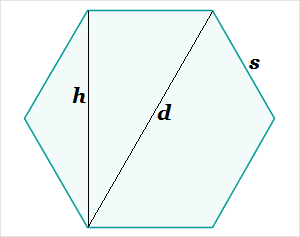# Hexagon Measurement Calculator

Hexagon Measurement Calculator
s =
h =
d =
area =
perimeter =
Select the radio button for one of the dimensions, enter its value, and click the calculate button to obtain the other measurementsThe side length, height, diagonal, and area of a regular hexagon are all interrelated. If you know the measure of one dimension, you can determine the others. For example, if the side length is s, the height h, the diagonal d, the area a, and the perimeter p, then you can calculate the other quantities with the following formulas:

h = (√3)s

d = 2s

a = (1.5√3)s²

p = 6s

Similarly,

s = h/(√3)

d = 2h/(√3), etc.

You can perform these computations using a hand calculator, our online scientific calculator, or the convenient hexagonal measurement calculator at left. All results are accurate within 5 decimal places.